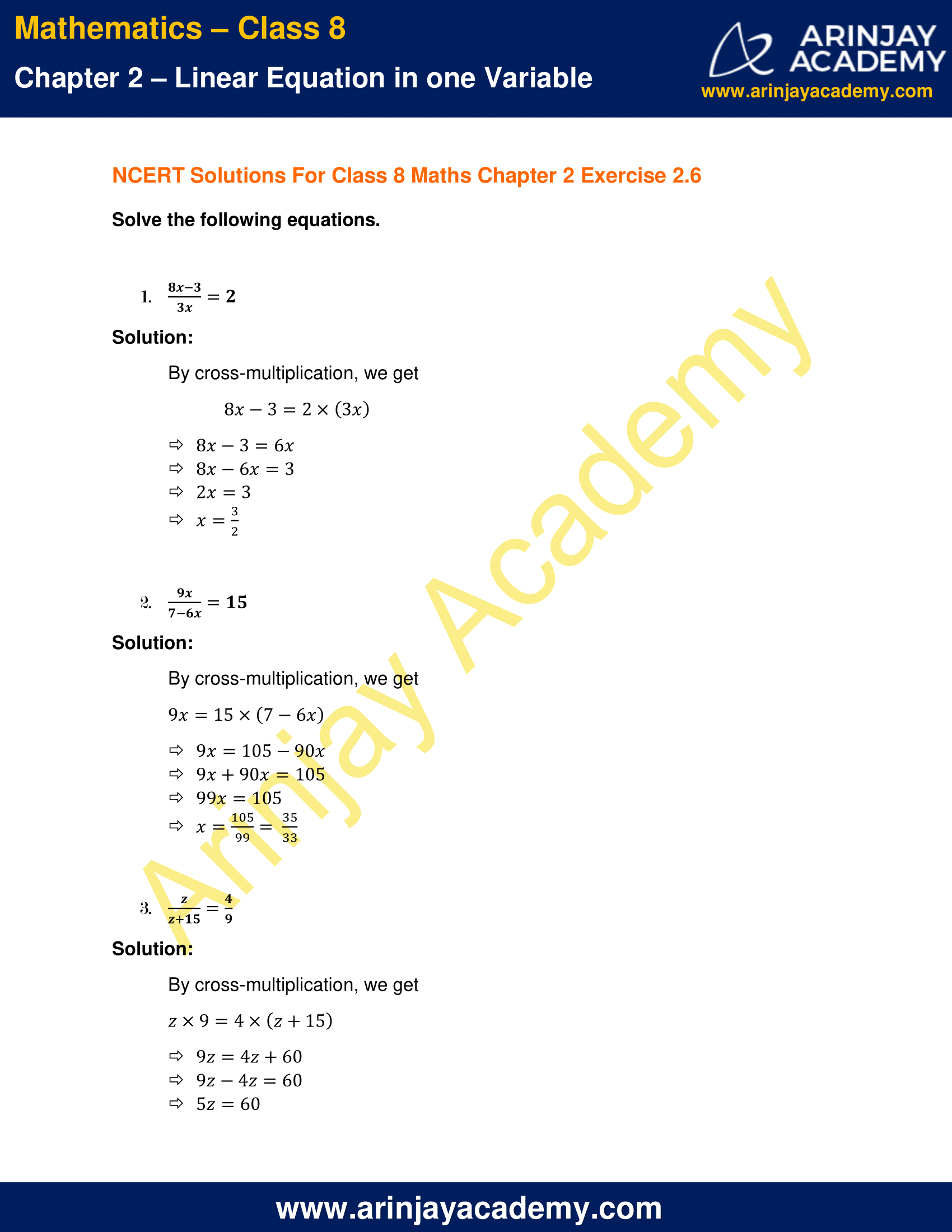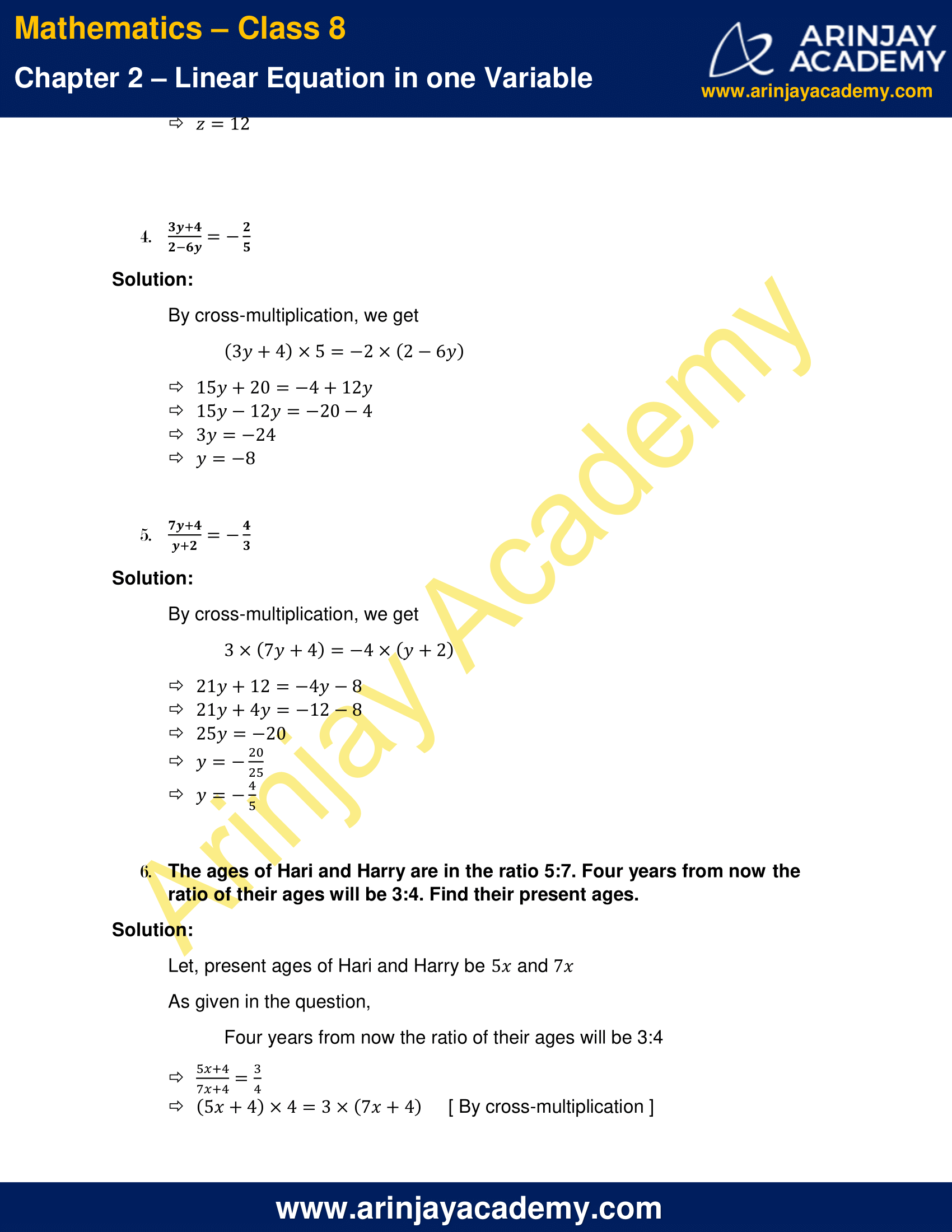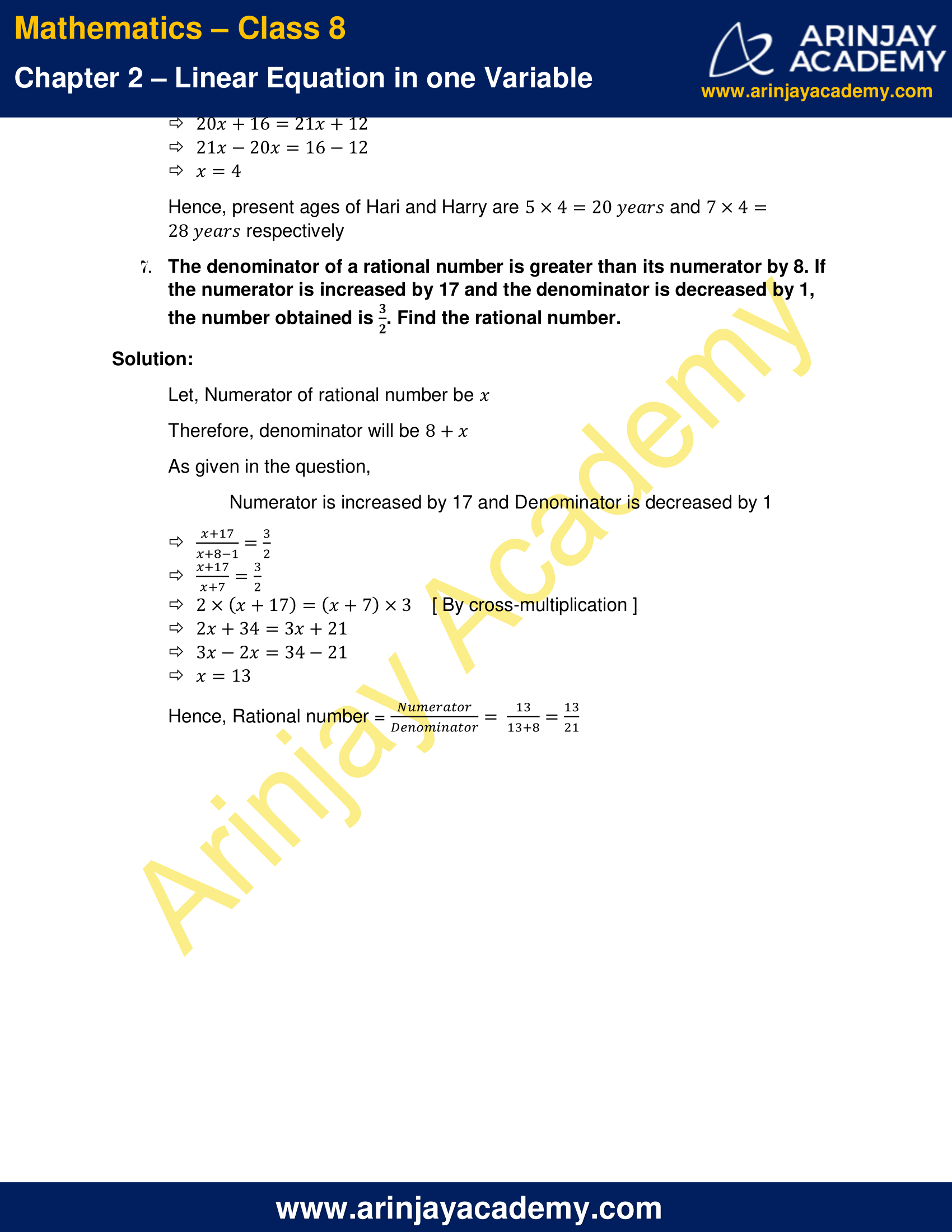# NCERT Solutions for Class 8 Maths Chapter 2 Exercise 2.6

NCERT Solutions for Class 8 Maths Chapter 2 Exercise 2.6 – Linear Equation in one variable, has been designed by the NCERT to test the knowledge of the student on the topic – Equations Reducible to the Linear Form

### NCERT Solutions for Class 8 Maths Chapter 2 Exercise 2.6NCERT Solutions for Class 8 Maths Chapter 2 Exercise 2.6

Solve the following equations.

1. (8x-3/3x) = 2

Solution:

By cross-multiplication, we get
8x – 3 = 2 × 3x
8x – 3 = 6x
8x – 6x = 3
2x = 3
x = 3/2

2. (9x/7-6x) = 15

Solution:

By cross-multiplication, we get
9x = 15(7 – 6x)
9x=105 – 90x
9x + 90x = 105
99x = 105
x = (105/99) = (35/33)

3. (z/z+15) = 4/9

Solution:

By cross-multiplication, we get
z x 9 = 4(z + 15)
9z = 4z + 60
9z – 4z = 60
5z = 60
z = 60/5
z = 12

4. (3y+4/2-6y) = -(2/5)

Solution:

By cross-multiplication, we get
(3y+4)x5 = -2x(2-6y)
15y+20=-4+12y
15y-12y=-20-4
3y=-24
y = (-24/3)
y=-8

5. (7y+4/y+2) = -(4/3)

Solution:

By cross-multiplication, we get
3 x (7y+4) = -4 x (y+2)
21y + 12 = -4y – 8
21y + 4y = -12 – 8
25y = -20
y = -20/25
y = -4/5

6. The ages of Hari and Harry are in the ratio 5:7. Four years from now the ratio of their ages will be 3:4. Find their present ages.

Solution:

Let, present ages of Hari and Harry be 5x and 7x
As given in the question,
Four years from now the ratio of their ages will be 3:4
(5x+4/7x+4) =  3/4
(5x+4) × 4 = 3 × (7x+4)
[ By cross-multiplication ]
20x + 16 = 21x + 12
21x – 20x = 16 – 12
x = 4
Hence, present ages of Hari and Harry are 5 x 4 = 20 years and 7 x 4 = 28 years respectively

7. The denominator of a rational number is greater than its numerator by 8. If the numerator is increased by 17 and the denominator is decreased by 1, the number obtained is . Find the rational number.

Solution:

Let, Numerator of rational number be x
Therefore, denominator will be 8+x
As given in the question,
Numerator is increased by 17 and Denominator is decreased by 1
(x+17/x+8-1) = 3/2
(x+17/x+7) = 3/2
[ By cross-multiplication ]
2 × (x+17) = (x+7) × 3
2x + 34 = 3x + 21
3x – 2x = 34 – 21
x = 13
Hence, Rational number = (Numerator/Denominator) = (13/13+8) = (13/21)

The next Exercise for NCERT Solutions for Class 8 Maths Chapter 3 Exercise 3.1 – Understanding Quadrilaterals can be accessed by clicking here